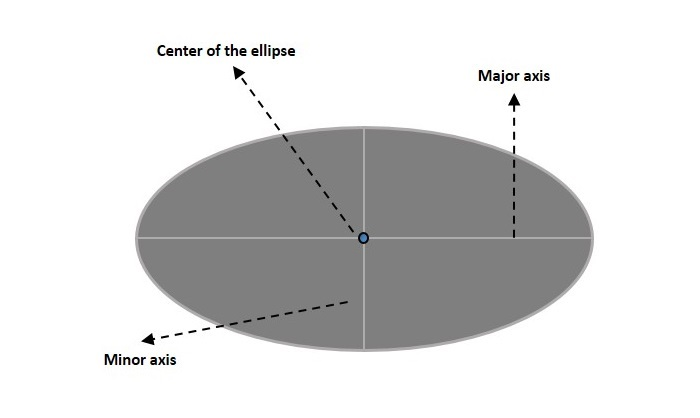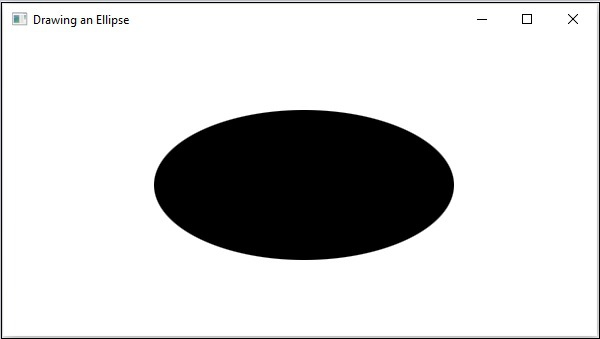# JavaFX - 2D Shapes Ellipse

An Ellipse is defined by two points, each called a focus. If any point on the Ellipse is taken, the sum of the distances to the focus points is constant. The size of the Ellipse is determined by the sum of these two distances. The sum of these distances is equal to the length of the major axis (the longest diameter of the ellipse). A circle is, in fact, a special case of an Ellipse.

An Ellipse has three properties which are−

• Centre − A point inside the Ellipse which is the midpoint of the line segment linking the two foci. The intersection of the major and minor axes.

• Major axis − The longest diameter of an ellipse.

• Minor axis − The shortest diameter of an ellipse.In JavaFX, an Ellipse is represented by a class named Ellipse. This class belongs to the package javafx.scene.shape.

By instantiating this class, you can create an Ellipse node in JavaFX.

This class has 4 properties of the double datatype namely −

• centerX − The x coordinate of the center of the ellipse in pixels.

• centerY − The y coordinate of the center of the ellipse in pixels.

• radiusX − The width of the ellipse pixels.

• radiusY − The height of the ellipse pixels.

To draw an ellipse, you need to pass values to these properties, either by passing them to the constructor of this class, in the same order, at the time of instantiation, as shown below −

```Circle circle = new Circle(centerX, centerY, radiusX, radiusY);
```

Or, by using their respective setter methods as follows −

```setCenterX(value);
setCenterY(value);
```

## Steps to Draw Ellipse

Follow the steps given below to draw an Ellipse in JavaFX.

### Step 1: Creating a Class

Create a Java class and inherit the Application class of the package javafx.application and implement the start() method of this class as shown below.

```public class ClassName extends Application {
@Override
public void start(Stage primaryStage) throws Exception {
}
}
```

### Step 2: Creating an Ellipse

You can create an Ellipse in JavaFX by instantiating the class named Ellipse which belongs to a package javafx.scene.shape. You can instantiate this class as follows.

```//Creating an Ellipse object
Ellipse ellipse = new Ellipse();
```

### Step 3: Setting Properties to the Ellipse

Specify the x, y coordinates of the center of the Ellipse → the width of the Ellipse along x axis and y axis (major and minor axises), of the circle by setting the properties X, Y, RadiusX and RadiusY.

This can be done by using their respective setter methods as shown in the following code block.

```ellipse.setCenterX(300.0f);
ellipse.setCenterY(150.0f);
```

### Step 4: Creating a Group Object

In the start() method, create a group object by instantiating the class named Group, which belongs to the package javafx.scene.

Pass the Ellipse (node) object created in the previous step as a parameter to the constructor of the Group class. This should be done in order to add it to the group as shown in the following code block −

```Group root = new Group(ellipse);
```

### Step 5: Creating a Scene Object

Create a Scene by instantiating the class named Scene which belongs to the package javafx.scene. To this class pass the Group object (root) created in the previous step.

In addition to the root object, you can also pass two double parameters representing height and width of the screen along with the object of the Group class as follows.

```Scene scene = new Scene(group ,600, 300);
```

### Step 6: Setting the Title of the Stage

You can set the title to the stage using the setTitle() method of the Stage class. The primaryStage is a Stage object which is passed to the start method of the scene class as a parameter.

Using the primaryStage object, set the title of the scene as Sample Application as follows.

```primaryStage.setTitle("Sample Application");
```

### Step 7: Adding Scene to the Stage

You can add a Scene object to the stage using the method setScene() of the class named Stage. Add the Scene object prepared in the previous step using this method as follows.

```primaryStage.setScene(scene);
```

### Step 8: Displaying the Contents of the Stage

Display the contents of the scene using the method named show() of the Stage class as follows.

```primaryStage.show();
```

### Step 9: Launching the Application

Launch the JavaFX application by calling the static method launch() of the Application class from the main method as follows.

```public static void main(String args[]){
launch(args);
}
```

### Example

Following is a program which generates an Ellipse using JavaFX. Save this code in a file with the name EllipseExample.java.

```import javafx.application.Application;
import javafx.scene.Group;
import javafx.scene.Scene;
import javafx.stage.Stage;
import javafx.scene.shape.Ellipse;

public class EllipseExample extends Application {
@Override
public void start(Stage stage) {
//Drawing an ellipse
Ellipse ellipse = new Ellipse();

//Setting the properties of the ellipse
ellipse.setCenterX(300.0f);
ellipse.setCenterY(150.0f);

//Creating a Group object
Group root = new Group(ellipse);

//Creating a scene object
Scene scene = new Scene(root, 600, 300);

//Setting title to the Stage
stage.setTitle("Drawing an Ellipse");

stage.setScene(scene);

//Displaying the contents of the stage
stage.show();
}
public static void main(String args[]){
launch(args);
}
}
```

Compile and execute the saved Java file from the command prompt using the following commands.

```javac EllipseExample.java
java EllipseExample
```

On executing, the above program generates a JavaFX window displaying an ellipse as shown below.javafx_2d_shapes.htm